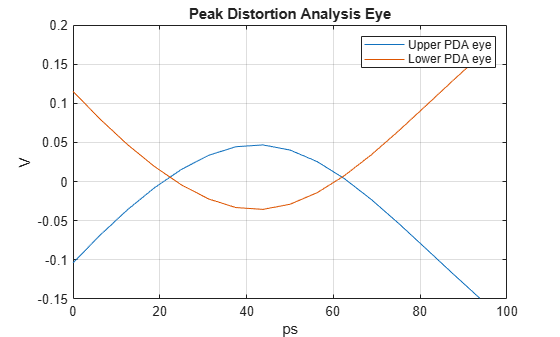# pulse2pda

Peak distortion analysis eye from pulse response

## Syntax

``[E,TH,D] = pulse2pda(P,N,M)``
``[E,TH,D] = pulse2pda(___,DC)``

## Description

example

````[E,TH,D] = pulse2pda(P,N,M)` calculates the peak distortion analysis (PDA) eye from a pulse response `P` with `N` samples per symbol and `M` levels of modulation.```
````[E,TH,D] = pulse2pda(___,DC)` also maintains the DC offset present in the pulse response `P` if `DC` is set to `true`.```

## Examples

collapse all

Load the pulse response column matrix from a file.

`load('PulseResponseReflective100ps.mat');`

Use the nonreturn to zero (NRZ) modulation scheme. Disregard any DC offset present in the pulse response.

```M = 2; DC = false;```

Calculate and plot the peak distortion analysis (PDA) eye.

```[pdaeye,th] = pulse2pda(pulse,SamplesPerSymbol,M,DC); figure t = th*SymbolTime*1e12; plot(t,pdaeye) legend('Upper PDA eye','Lower PDA eye') xlabel('ps') ylabel('V') title('Peak Distortion Analysis Eye') grid on```## Input Arguments

collapse all

Input pulse response, specified as a column matrix. The first column contains the primary pulse response and the subsequent columns (if any) contain the crosstalk pulse responses.

Data Types: `double`

Number of samples per symbol, specified as a positive integer scalar.

Data Types: `double`

Number of modulation levels, specified as a positive integer scalar. `M` defines the modulation scheme used in the peak distortion analysis (PDA) calculation.

• If `M` = 2, the modulation scheme is nonreturn to zero (NRZ).

• If `M` = 4, the modulation scheme is four-level pulse amplitude modulation (PAM4).

Data Types: `double`

Determine whether to maintain the DC offsets in pulse response `P`.

• If `DC` is set to `true`, the `pulse2pda` function maintains the DC offsets present in the pulse response.

• If `DC` is set to `false`, the `pulse2pda` function disregards the DC offsets present in the pulse response.

Data Types: `double`

## Output Arguments

collapse all

Peak distortion analysis (PDA) eye, returned as a vector.

Data Types: `double`

Horizontal time histogram bin centers, returned as a vector.

Data Types: `double`

Upper pattern of the PDA eye limit, returned as a vector.

Data Types: `double`